# 2nd PUC Basic Maths Question Bank Chapter 15 Circles Ex 15.1

Students can Download Basic Maths Exercise 15.1 Questions and Answers, Notes Pdf, 2nd PUC Basic Maths Question Bank with Answers helps you to revise the complete Karnataka State Board Syllabus and score more marks in your examinations.

## Karnataka 2nd PUC Basic Maths Question Bank Chapter 15 Circles Ex 15.1

Part – A

2nd PUC Basic Maths Circles Ex 15.1 One Mark Questions and Answers

Question 1.
Find the equation of the circle with centre ‘C’ and radius ‘r’ in each of the following:
(a) c(-3, 2) and r= 5 units
(b) c(0, 0) and r = 4 units
(c) c(-1,-2) and diameter = 25 units
(d) c(1, 1) and r = $$\sqrt{2}$$ units
(e) c(a cos θ, b sin θ) and r = a units.
(a)Equation of the circle is(x – x1)2 + (y – y1)2 = r2
(x-(-3))2 + (y – 2)2 = 52
(x + 3)2 + (y – 2)2 = 52
x2 + y2 + 6x – 4y – 12 = 0.

(b) Given C = (0,0) & r = 4 units
The equation of the circle is
(x – 0)2 + (y – 0)2 = 42
⇒ x2 + y2 = 16(c) C = (-1,-2 )& r = $$\frac{\text { diameter }}{2}=\frac{25}{2}$$
The equation of the circle is
(x + 1)2 + (y + 2)2 = $$\left(\frac{25}{2}\right)^{2}$$
x2 + 2x + 1 + y2 + 4y +4 = $$\frac { 625 }{ 4 }$$
⇒ 4x2 + 4y2 + 8x + 16y – 605 = 0.

(d) C = (1, 1) r = $$\sqrt{2}$$ units
Equation of the circle is
(x – 1)2 +(y – 1)2 = $$(\sqrt{2})^{2}$$
⇒ x2 + 1 – 2x + y2 + 1 – 2y = 2
⇒ x2 + y2 – 2x – 2y = 0

(e) C = (a cos θ, a sin θ) and r = a units
Equation of the circle is (x – a cos θ)2+ (y – a sin θ)2 = a2
⇒ x2 + a2 cos2 θ – 2a x cos θ + y2 + a2 sin2 θ – 2a y sin θ = a2
⇒ x2 + y2 – 2a x cos θ – 2 ay sin θ + a2 (cos2 θ + sin2 θ) = a2
⇒ x2 + y2 – 2a x cos θ – 2a y sin θ + a2 – a2= θ
⇒ x2 + y2 – 2a x cos θ – 2a y sin θ = 0 (∵  cos2 θ+ sin2 θ = 1)

Question 2.
Find the centre of the circle, two of the diameters are
(a) x + y = 2, x – y = 0
(b) 2x – 3y = 1, 3x – 2y = 2
(c) y = 0 and y = x – 5
(a) Given x + y = 2, x – y = 0 solving these two equations we get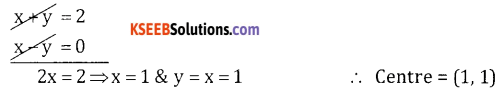(b) Given 2x – 3y = 1 → × 3
3x – 2y = 2 → × 2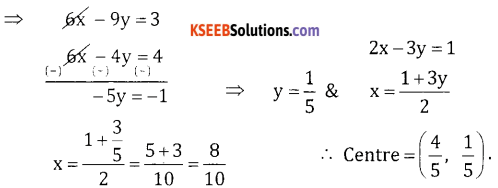(c) y = 0 ⇒ 0 = x – 5 ⇒ x = 5
∴ centre = (5,0).Question 3.
Write the equation of the point circle with centre at
(a) (4, -5)
(b) (-3, 2)
(c) (1,0).
(a) Point circle means circle whose radius = 0
Given C = (4,-5) & r = 0
The point circle & (x – 4)2 + (y + 5)2 = 0
⇒ x2 + y2 – 8x + 10y + 41 = 0

(b) Centre (-3,2)
The point circle is (x + 3)2 + (y – 2)2 = 0
⇒ x2 + y2 + 6x – 4y + 13 = 0

(C) Centre (1,0)
∴ Circle is (x – 1)2 + (y – 0)2 = 0
x2 – 2x + 1 + y2 = 0 ⇒ x2 + y2 – 2x + 1 = 0.

Part -B

2nd PUC Basic Maths Circles Ex 15.1 Two Marks Questions and Answers

Question 1.
Find the equation of the circle.
(a) Two of the diameters are x + y = 6 and x + 2y = 4 and its radius is 10 units.
(b) Two of the diameters x + y = 4 and x – y = 2 and passing through the point (2,-1).
(c) Two of the diameters are 2x – 3y = 5 and 3x – 4y = 7 are the diameters of a circle of area 154 Sq. cm.
(a) Given r = 10, & Diameters are x + y = 6 & x + 2y = 4
Solving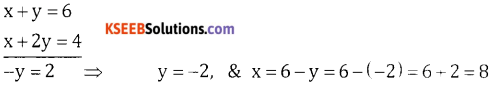∴ Centre = (8,-2) & r = 10 units.
∴ Equation of the circle is (x – 8)2 + (y + 2)2 = 10
⇒ x2 + y2 – 16x + 4y – 32 = 0.(b) Point of inter section of two diameters x + y = 4 & x – y = 2 is the centre.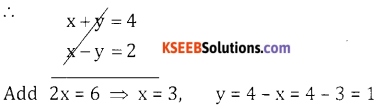∴ Centre C = (3, 1). Also given P(2, – 1)
r = CP = $$\sqrt{(2-3)^{2}-(-1-1)^{2}}=\sqrt{(-1)^{2}+(-2)^{2}}=\sqrt{1+4}=\sqrt{5}$$
∴ The required equation of the circle with centre (3, 1) & radius = $$\sqrt{5}$$ is
(x – 3)2 – (y – 1)2 = $$(\sqrt{5})^{2}$$
x2 + 9 – 6x + y2 + 1 – 2y = 5
⇒ x2 + y2 – 6x – 2y + 5 = 0.

(c) Point of intersection of the two diameters is centre
2x – 3y = 5 → × 3
3x – 4y = 7 → × 2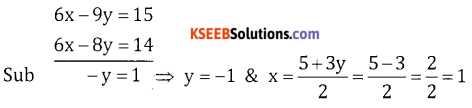∴ C = (1, – 1)
Also Given Area of the circle = 154 sq. cm
⇒ πr2 = 154
$$r^{2}=\frac{154}{\pi}=\frac{154}{22} \times 7=49$$
⇒ r = 7
∴ Required Equation of the circle with centre (1, -1) & r = 7 units is
(x – 1)2 + (y + 1)2 = 49
x2 + 1 – 2x + y2 + 2y + 1 = 49
x2 + y2 – 2x + 2y – 47 = 0.

Question 2.
Find the equation of the circle with centre at (-2, 1) and passing through the origin.
Given C = (-2, 1) & Let P = (0,0)
r = CP = $$\sqrt{(0-(-2))^{2}+(0-1)^{2}}=\sqrt{2^{2}+(-1)^{2}}=\sqrt{4+1}=\sqrt{5}$$
∴ Required equation of the circle with centre C = (-2, 1) & r = $$\sqrt{5}$$ units is
(x + 2)2 + (y – 1)2 = $$(\sqrt{5})^{2}$$
⇒ x2 + y2 + 4x – 2y = 0.Question 3.
Find the equation of the circle with centre at (2, 1) and passing through (0, -1).
Given Centre = C (2, 1) & Let P = (0, -1)
Here r = CP = $$\sqrt{(0-2)^{2}+(-1-1)^{2}}=\sqrt{4+4}=\sqrt{8}$$ units
Equation of the circle with centre (2, 1) & r = $$\sqrt{8}$$ is
(x – 2)2 + (y – 1)2 = $$(\sqrt{8})^{2}$$
x2 + 4x + y2 + 1 – 2y = 8
x2 + y2 – 4x – 2y – 3 = 0.

Question 4.
Find the equation of the circle described on the line joining the points A and B as diameter where.
(a) A(-5, 1) and B(1,3)
(b) A(2,0) and B(0, 2)
(c) A(3,4) and B(1, -2)
(a)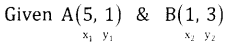Equation of the circle is (x – x1) (x – x2) + (y – y1) (y – y2) = 0
i.e., (x + 5) (x – 1) + (y – 1) (y – 3) = 0
⇒ x2 + 5x – x – 5 + y2 – 3y – y + 3 = 0
⇒ x2 + y2 + 4x – 4y – 2 = 0.

(b)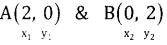Equation of the circle is (x – x1)(x – x2) + (y – y1) (y – y2) = 0
i.e., (x – 2)(x – 0) + (y – 0) (y – 2) = 0
x2 – 2x + y2 – 2y = 0
⇒ x2 + y2 – 2x – 2y = 0

(c) Given A(3,4) B(1, -2)
∴ Required equation is (x – x1)(x – x2) + (y – y1) (y – y2) = 0
i.e., (x – 3) (x – 1) + (y – 4) (y + 2) = 0
x2 – x – 3x + 3 + y2 + 2y – 4y – 8 = 0
⇒ x2 + y2 – 4x – 2y – 5 = 0.error: Content is protected !!